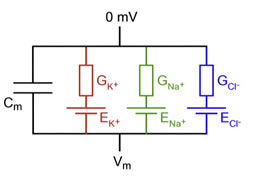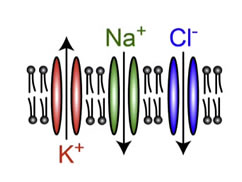# Ionic Current current flowThis section covers the cell analogy of an electrical circuit with resistor and capacitor components.

An electrical equivalent of a cell with three ion channels can be represented as follows:Where:

• Cm is the membrane capacitance formed by the lipid bilayers
• Gk+ is the Potassium conductance (1/Resistance)
• Ek+ is the reversal potential of Potassium (from the Equilibrium Table) -97 mV)
• GNa+ is the Sodium conductance
• ENa+ is the reversal potential of Sodium +67 mV
• GCi- is the Chloride conductance
• ECi- is the reversal potential of Chloride -85 mV

Using Ohm's law:

$V = I R I = V G$

Where:

• V=Voltage across the ion channel
• I=Ionic current through the channel
• R=Resistance to the flow
• G=The conductance

Since R is 1/conductance we can rewrite the equation to be

I=VG

Capacitance:

$Q = C V I = C d V d T$

where:

• Q = the charge in the capacitor
• C = the capacitance
• V=The voltage across the capacitor

We know that the current (I) is equal to the rate of change of the charge. Differentiated to give the capacitive current flow.

Therefore the total trans-membrane current (Im)consists of four current flows.

$I m = I c + I k + + I N a + + I C I − I c = C m d V m d t I k + = ( V m − E k + ) G k + I N a + = ( V m − E N a + ) G N a + I C I − = ( V m − E C I − ) G C I −$

So to change the membrane potential, we have to change the ionic conductances for Potassium, Sodium or Chloride.

For example: What happens to Vm if the Potassium channel (K+) Opens?

The Potassium ions will escape the inside of the cell membrane and move down their concentration gradient to the outside leaving a negative charge inside the cell. This causes the membrane potential to move toward the Potassium reversal potential (about -90 mV).The complete voltage across the capacitor can be modeled with the RC time constant equation:

## $V m = E k ( 1 − e − t / R C ) )$

The term RC is the membrane time constant. If the cell has a high resistance (low conductance), then the cell will take a long time to change. If the capacitance is high, it will also take a long time to change.

The equation also shows us that the membrane cannot change instantaneously - it changes on the scale of RC.

The equivalent schematic of the cell with only the Potassium channel is:## Opening a Channel

The opening of the ion channels in a specific order has the following effect.This is the basis of ion channel dynamics.